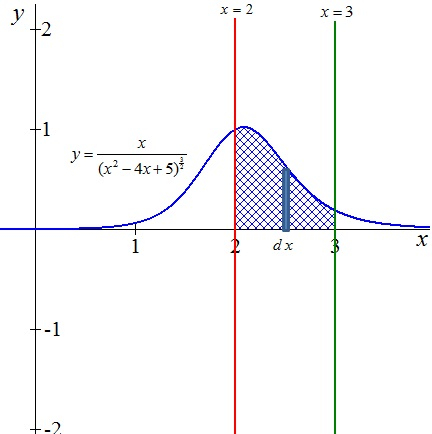Find the area bounded by the graph of y = \frac{x}{(x^2 - 4x + 5)^\frac{3}{2}} and the x-axIs...

Question:

Find the area bounded by the graph of {eq}y = \frac{x}{(x^2 - 4x + 5)^\frac{3}{2}} {/eq} and the x-axIs for {eq}2 \leq x \leq 3. {/eq}

Calculus of Area of a Region using Integrals:

The area of a region with known geometry can be determined from formulas, however, when it comes to the area of a region limited by curves, it is necessary to use integration and the fundamental theorem of the calculus.

Definite integral as area of a region:

If {eq}\displaystyle f(x) {/eq} is continuous and not negative in the closed interval {eq}\displaystyle [a,b] {/eq}, then the area of the region bounded by the graph of {eq}\displaystyle f(x) {/eq}, the {eq}\displaystyle x {/eq}-axis and the vertical lines {eq}\displaystyle x=a {/eq} and {eq}\displaystyle x=b {/eq} is given by

{eq}\displaystyle A=\int_a^bf(x)dx {/eq}

The region is shown in the figure below:Area of the region

The required area is calculated using the formula

{eq}\displaystyle A= \int_ a ^ b [ f ( x ) - ( g ( x ) ]d x {/eq}

Data.

Function: {eq}f(x)= {\frac {x}{ \left( {x}^{2}-4\,x+5 \right) ^{3/2}}} {/eq}

Lower limit of integration: {eq}a= 2 {/eq}

Upper limit of integration: {eq}b= 3 {/eq}

{eq}\displaystyle A = \displaystyle \int_{ 2 }^{ 3 } \left( {\frac {x}{ \left( {x}^{2}-4\,x+5 \right) ^{3/2}}} \right)dx \\ {/eq}

Solving the integral using a Table of Integrals:

{eq}\displaystyle A = \displaystyle \left( {\frac {2\,x-5}{\sqrt {{x}^{2}-4\,x+5}}} \right) \vert_{ 2 }^{ 3 } \\ {/eq}

Applying the fundamental theorem of calculus:

{eq}\displaystyle A = \displaystyle \left[ {\frac {2\,(3)-5}{\sqrt {{(3)}^{2}-4\,(3)+5}}} \right] - \left[ {\frac {2\,(2)-5}{\sqrt {{(2)}^{2}-4\,(2)+5}}} \right] \\ \displaystyle A = \displaystyle \frac{1}{2}\,\sqrt {2} - \left( -1 \right) \\ \displaystyle A = \displaystyle \frac{1}{2}\,\sqrt {2}+1 {/eq}